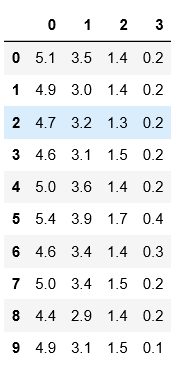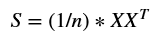# Principal Component Analysis : Dimensionality Reduction Technique — Step by Step Approach

Before analyzing data and coming down to some inferences, it is often necessary to visualize the data set, in order to get an idea of it. But, nowadays data sets contain a lot of random variables (also called features) which become difficult in visualizing the data set. Sometimes it is even impossible to visualize such high dimensional data. Here is where we come across dimensionality reduction. As the name suggests it is —

The process of reducing the number of random variables of the data set under consideration, via obtaining a set of principal variables.

Easy enough? Great.

In order to reduce the number of random variables, we answer the first question — which random variables are we going to remove? Tough question! Because removing any variables randomly may lead to information loss and hence lose the sole purpose of the data set.

This is where dimensionality reduction techniques come to rescue. Broadly, dimensionality reduction has two classes — feature elimination and feature extraction.

Feature elimination is removing some variables completely if they are redundant with some other variable or if they are not providing any new information about the data set. The advantage of feature elimination is that it is simple to implement and makes our data set small, including only variables in which we are interested. But as a disadvantage — we might lose some information from the variables which we dropped.

Feature extraction, on the other hand, is the formation of new variables from the old variables. Say, you have 20 variables in your data set, then feature extraction technique will create 20 new variables which are combinations of 20 old variables. PCA is the example of one such feature extraction method.

### PCA — Principal Component Analysis

If we make 20 new variables, then where we have reduced its dimension? To reduce dimension, we consider the 20 variables and take the ones that give us maximum variance across the data sets. Let us see these details by implementing PCA step by step (without using inbuilt package) —

We will start with the small data set — Iris data set. The Iris data set contains 150 rows describing the measurements of flowers belonging to three different species. Three classes of the data set are — Iris-setosa (n=50), Iris-versicolor (n=50), Iris-virginica (n=50). It has 4 features which are measurements of sepal and petal — sepal width, sepal height, petal width and petal height.

#### 1. Import the required libraries

For matrix calculations, we will use numpy package for Python.

`import numpy as np  import matplotlib.pyplot as pltimport pandas as pd frame`

#### 2. Let’s first read the data set and store it in a data frame

The following code prints the first 10 rows of the data set.

`file_name = 'https://archive.ics.uci.edu/ml/machine-learning-databases/iris/iris.data'df = pd.read_csv(file_name, sep=',',header=None)df.head(n=10)`Result for the above code snippet — Dimensions: 150 X 5

As we can see in the results of top 10 rows of the data set, there are 4 columns (features of the data set) and one class column (last column). We will reduce the dimensionality of this data set from 4D to 2D.

#### 3. Extract the last column (class label column)

We will extract the last column of the data set, and store it in a new data frame.

`class_label = pd.DataFrame(df.iloc[:,-1])class_label.columns = ['label']df = df.iloc[:, :-1]df.head(n=10)`Result for the above code snippet- Dimensions: 150 X 4

Now we have two data frames — one for features and another one for class label.

#### 4. Normalizing the data

This is one of the most important steps in PCA. It makes sure that your end result is not dominated by a single variable. So we have to standardize the data set, as it is possible that different variables are measured in different scales. Mean normalization is implemented by taking mean of every column, and then subtracting the mean vector from every row/record. The resultant data set is the normalized data set. This is implemented in one step — shown below:

`df = df.sub(df.mean(axis=0), axis=1)`

#### 5. Calculating covariance

For mathematical calculations, we will first transform our features data frame into a matrix, and then calculate covariance on feature matrix. The formula for covariance is —Covariance formula- Dimensions: 4 X 4

In the above formula, X is the data matrix and n is the number of rows in the data set. Also, numpy package has a built-in function for covariance as cov.

`df_mat = np.asmatrix(df)sigma = np.cov(df_mat.T)`

We have taken df_mat.T, as we are finding covariance among features of the data set. sigma will be our resultant covariance matrix with 4X4 dimensions.

#### 6. Finding eigen values and eigen vectors

We found the relationships among the features in the fifth step. Now, let us find the eigen values and eigen vectors of the covariance matrix sigma. This is also called an eigen decomposition. The eigen values tell us the variance in the data set and eigen vectors tell us the corresponding direction of the variance. The following code snippet finds the eigen values and eigen vectors —

`eigVals, eigVec = np.linalg.eig(sigma)`

From the above formula, we will get 4 eigen values and the coresponding eigen vectors matrix of 4X4 dimensions.

#### 7. Sort the eigen values and eigen vectors

As we are interested in maximum variance across features, we will sort the eigen values in decreasing order and retrieve their corresponding eigen vectors accordingly.

`sorted_index = eigVals.argsort()[::-1] eigVals = eigVals[sorted_index]eigVec = eigVec[:,sorted_index]`

#### 8. Select top k eigen values and corresponding eigen vectors

To reduce dimensions of the data set from d features to k features, we select the top k eigen values and the corresponding eigen vectors (in our case k=2, as we are reducing data set to 2 dimensions). We require only the eigen vectors for our further calculations and hence we will store it in the eigVec variable.

`eigVec = eigVec[:,:2]`

#### 9. Forming the new data set in reduced dimensions

As we have the top 2 eigen vectors and the original matrix with us, its time to form the new data set with reduced k-dimensions (here k=2). PCA being a linear technique, it forms linear equation between the old data set and new data set.

new data set =dot product([old data set],[eigen vector] )

The following code snippet will show you the implementation of this step —

`transformed = df_mat.dot(eigVec)`

Let us attach the class label to this new data set and form a new data frame which will be ready to plot.

`#horizontally stack transformed data set with class label.final_df = np.hstack((transformed, class_label))`
`#convert the numpy array to data framefinal_df = pd.DataFrame(final_df)`
`#define the column namesfinal_df.columns = ['x','y','label']`

The above code makes the final_df data frame of the dimensions: 150 X 3, which has three columns — x, y, label.

#### 10. Plot the new data set

`groups = final_df.groupby('label')figure, axes = plt.subplots()axes.margins(0.05)for name, group in groups:    axes.plot(group.x, group.y, marker='o', linestyle='', ms=6, label=name)    axes.set_title("PCA on pca_a.txt")axes.legend()plt.xlabel("principal component 1")plt.ylabel("principal component 2")plt.show()`Scatter plot of PCA on IRIS data set.

Feel free to view the code on GitHub link

#### Happy coding!